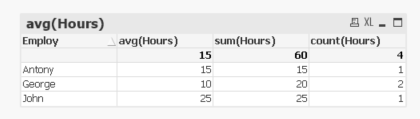QlikView App Development

Discussion Board for collaboration related to QlikView App Development.

Contributor

Trendline AVG Calculation

Hello,

the attached example shows the worked hours made by some employees; in the chart is shown the calculated AVG (the green reference line) and the Average trend line (the red one).

The sum of the hours is 60 (according to the hours line of the graph), so the right result for the average is 15 (as shown by the AVG formula).

Could be someone so kind to explain me the average 20 result by the trendline calculation?

Thank you.

N.

1 Solution

Accepted SolutionsMVP

Re: Trendline AVG Calculation

I know you are not satisfied with the average which is calculated, but that is how it is... to read more on the topic of averages, you can read here:

Average – Which average?

15 RepliesPartner

Re: Trendline AVG Calculation

Hi Nicola,

The problem is that George has 2 imputations of 10 hours. So the avg is (10 + 10 + 20+ 20)/4.

What you need is this: AVG(aggr(sum(Hours),Employ)).Regards,

Sorin.

Contributor

Re: Trendline AVG Calculation

Hi Sorin,

thank you for the prompt replay; the average is (25+15+10+10)=60/4=15, in fact my question was about the wrong average trendline which shows 20, not about the AVG formula shown in the reference one (which shows 15, correctly).

N.MVP

Re: Trendline AVG Calculation

May be use an expression to create a line for average may be

=Sum(TOTAL Hours)/Count(TOTAL Employ)

Contributor

Re: Trendline AVG Calculation

Thank you for the reply, Sunny,

your formula gives 15 as result (and It is the right result...I Know it).

It seems I'm not able to explain what is my question....I'd like to know if anybody can explai to me the formula used by QV to calculate the Average Trendline because it seems wrong to me.

Thank you.

N.

Highlighted
Valued Contributor II

Re: Trendline AVG Calculation

I think it's summing the hours which equals 60.  And dividing by unique employs which is 3.

60/3 = 20.

I think using a trendline is erroneous when you have that kind of dimension.  typically you would use a trendline over a chronological time period.

actually using a line chart is appropriate either.  you should probably use a bar chart.MVP

Re: Trendline AVG Calculation

I think QV is calculating it like this

Sum(Hours) = 60

Count(DISTINCT Employee) = 3

Sum(Hours)/Count(DISTINCT Employee) = 20

Contributor

Re: Trendline AVG Calculation

I agree with you, Wallo....but what kind of average is this?

Valued Contributor II